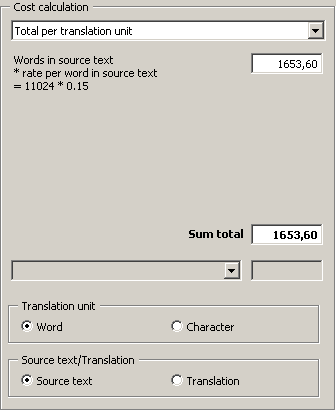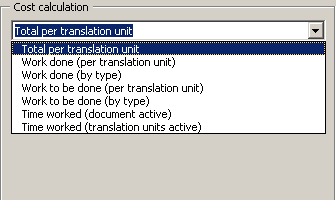Cost calculation

This tab contains cost calculations based upon the statistical information retrieved.The frame consists of three elements:

·       You can select the calculation type in the drop-down box located at the top.

·       The calculation results, and some average figures (if appropriate, see below), are shown in the Calculation frame located below.

·       In the Translation unit and Source text/Translation frames, you can select the units used for the calculation (active, if appropriate).

These are the available calculation types:·       Total per translation unit:

This is the simplest and most widely used calculation for the complete costs of a translation. It has become the worldwide standard for calculating translation costs.

Formula:

Number of words/characters in source text * rate per word/character in source text

Note: Average figures are not available for this calculation type because no meaningful values can be calculated.

·       Work done (per translation unit):

This is a simple way to measure the costs for the work done, based on the standard way to calculate translations costs (see above).

Formula:

Number of translated words/characters in source text/translation * rate per word/character in source text/translation

·       Work done (weighted, by type):

This is a more sophisticated way of calculating translation costs, increasingly used because, in some cases, the true translation costs are better reflected by this calculation if a translation memory was used for the translation. In MetaTexis, you can now measure the impact of a TM on the translation costs. Even if this impact cannot be measured perfectly, it is possible to make an approximation.

The formula consists of three elements:

Costs for the words/characters in the source text/translation which were translated manually or on the basis of terminology results;

Costs for the words/characters in the source text/translation which were translated by a translation machine.

Costs for the words/characters in the source text/translation that were translated on the basis of TM matches.

Formula:

Number of manually translated words/characters * price per word in source text/translation

+ Number of machine translated words/characters in source text/translation * revision rate per word/character in source text

+ Number of words/characters in source text/translation translated on the basis of TM matches * revision rate per word/character in source text/translation

·       Work to be done (per translation unit):

This is a simple way of measuring the costs for the work done, based on the standard way of calculating translations costs (see above).

Formula:

Number of translated words/characters in source text/translation * rate per word/character in source text

+ Number of words/characters in TUs to be edited * rate per word/character in source text

Note: Average figures are not available for this calculation type because no meaningful values can be calculated.

·       Work to be done (weighted, by type):

This calculation supplements the last option. It informs you (or your client) about the costs of the work to be done.

The formula consists of three elements:

Costs for the number of words to be translated

Costs for the number of words in TUs with TDB results

Costs for the number of words in TUs with TM matches

Formula:

Number of words/characters to be translated * rate per word in source text

+ Number of words/characters in TUs with TDB results to be edited * rate per word in source text

+ Number of words/characters in TUs with TM matches to be edited * revision rate per word/character

Note: Average figures are not available for this calculation type because no meaningful values can be calculated.

·       Time worked (document active):

MetaTexis measures the time worked on a document as precisely as possible. Therefore, you or your client gets reliable results if you calculate the translation costs on the basis of the time when the document was active.

Formula:

Time worked (document active) * price per hour time worked

·       Time worked (translation units active):

This calculation type is based on the time while translation units were active. It is less meaningful than the last type because you can revise the document even when no TU is active. This calculation type is mainly added for the sake of information.

Formula:

Time worked (translation units active) * price per hour time worked

Note: To make sure that the information about the time worked is correct, a screensaver should be active when doing a translation with MetaTexis. Moreover, the screensaver should be activated relatively quickly after a short time of idleness.